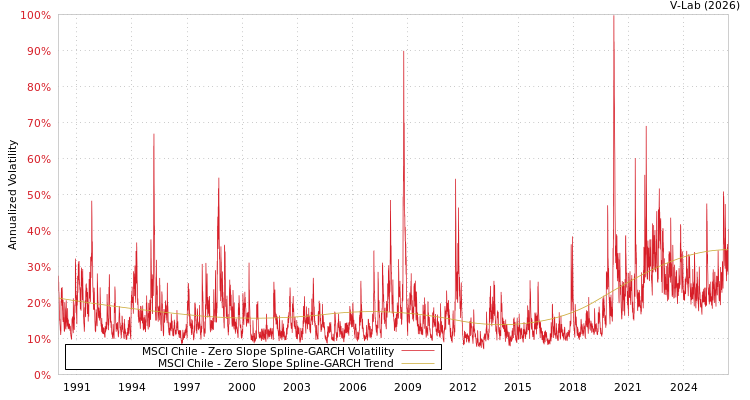##### MSCI Chile Zero Slope Spline-GARCH Volatility Analysis
###### Volatility Prediction for Friday, February 3rd, 2023:25.92% (-0.27%)

Analysis last updated: Friday, February 3, 2023, 05:06 PM UTC

###### Date Range:

from

to

6M ·

1Y ·

2Y ·

5Y ·

10Y ·

Allparamt-stat
$\omega$1.15724.70
$\alpha$0.134411.83
$\beta$0.820563.16
${\gamma }_{1}$0.00860.13
${\gamma }_{2}$0.02150.20
${\gamma }_{3}$-0.0594-0.78
${\gamma }_{4}$0.01120.19
${\gamma }_{5}$0.11162.19
${\gamma }_{6}$-0.2041-3.50
${\gamma }_{7}$0.17392.95
${\gamma }_{8}$-0.1056-1.92
${\gamma }_{9}$0.15863.13
${\gamma }_{10}$-0.2053-5.52
Estimation Period:
Jan 1, 1990 to Nov 25, 2022